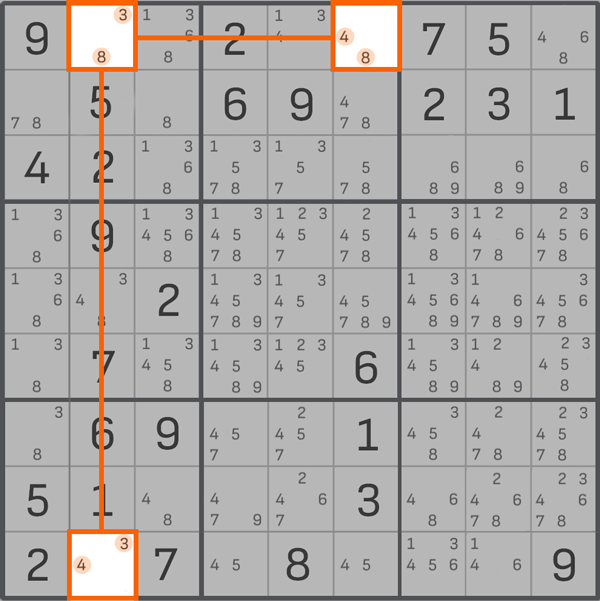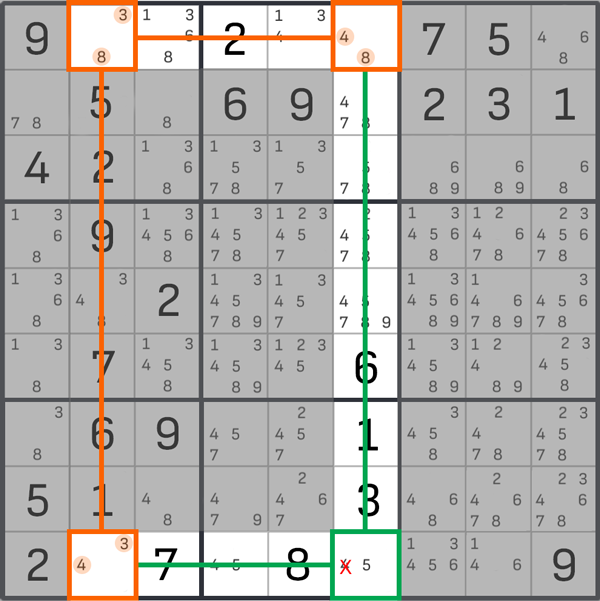# Y-wing - sudoku technique

"Y-Wing" is an advanced method of solving sudoku puzzles. It is suitable for people who have reached the expert level and choose hard sudoku levels.

The "Y-Wing" method is similar to the "X-Wing" method. But the difference is the number of corners used - 3 instead of 4.

Let's look at an example. Let's find a cell with only 2 notes, 3 and 8. This cell will be called "pivot".

Next, we need to find 2 more cells in which we have left 2 notes each - such cells are called "branches". The conditions for using this technique are that the "branches" must be in the same column, row, or block as the "pivot", and one of the 2 numbers in each "branch" must be the same as the one in the "pivot", and the second number must be the same for the "branches". In our example, these are the cells with the notes 4-8, 3-4 (here 3 and 8 are the same as the notes in the stem, and 4 is in both branches).

Now we need to see where the columns and rows of the branches intersect. This means we need to draw more lines (as in the example below).When we have mentally drawn lines and "outlined" the Sudoku field, we see the intersection cell at the bottom (it has two numbers - 4 and 5). We understand that a double is formed vertically and horizontally. In this case, we can remove the 4 from the intersection cell, leaving a 5. So the "Y-Wing" method has helped fill at least one cell (and we know exactly what a 5 should be in it). This simplifies the process of filling other blocks, columns, and rows in the Sudoku.